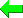Print Version Page Index
Solvers
Puzzles
Basic Strategies
Tough Strategies
Diabolical Strategies
Extreme Strategies
Deprecated Strategies
Str8ts
Other

# SK Loops

The reason SK Loops are in the 'diabolical' strategies and not the 'extreme' as this is quite a stand-out pattern. However, it was discovered (invented?) trying to solve the Easter Monster and it requires a crowded field of candidates, which is typical of the very hardest puzzles.

Credits first: I'm indebted to pjb or Phil from Sydney, Australia for his write up here and the many examples he's collected on his website here. The solver will find SK Loops for all Phil's types from the vanilla down to Type H, but not as yet "Related Loops". In my implementation I don't discern 'types' as I've generalized the detection. SK Loops are apparently a subset of something larger but I've not explored this yet.

I'm going to start, as Phil does, with the Easter Monster, since the pattern is nicely spread out and symmetrical. SK Loops are bi-directional continuous loops meaning that the loop can be started at any point and you can trace the links in either direction.

First find four solved or given cells spread over four boxes such that they make a rectangle. Phil distinguishes between givens (clues) and solved cells but I do not and I've not found a false positive because of this.

Shown in the diagram are four such numbers and surrounding them are eight 2-cell pairs containing Hidden Pairs
The pattern definition is then
• The loop requires 4 boxes in 2 bands and and 2 stacks, and 8 links, one link in each box, one link in each row and one link in each column.
• In each box the cell at the intersection of the row cells and column cells is a given.
• Loops can include single, double or triple links, as long as the sum of the links is less than or equal to 16.
• Of the 2 cells involved in a row or column within a box, one of the two can be solved, but not a given.
Since we have eight pairs of cells, sixteen numbers will be required to fill all those cells. The solver highlights candidates in green and blue. These are the alternative solutions. All sixteen cells will contain either the green numbers or the blue numbers, eventually.

Now, the pattern definition requires sum of the links is less than or equal to 16. To see what we are counting, here is the formal definition of the loop

(27=38)B13- (38=16)B79- (16=39)AC8- (39=27)GJ8- (27=45)H79- (45=16)H13- (16=48)GJ2- (48=27)AC2-

The solver always starts at the top-left most given cell and the row and loops clockwise, so pair B13 starts. (Phil's starts in a different place in his documentation). B13 is Strongly Linked to B79 on the Hidden Pair {3,8}. Looking at all the links and counting the candidates in each pair we get
SK Loop Type 2-2-2-2-2-2-2-2-
and those numbers add up to 16.

Since we have identified the content of these sixteen cells as a Locked Set we can eliminate along the unit of alignment. So, since B13 contains a blue {3,8} and B79 contains a green {3,8} and the solution will be one or the other, so we can remove 3 and 8 from B5 and B6.

This SK Loop alternates Triple and Single links instead of the classic doubles all round. This makes the type 3-1-3-1-3-1-3-1- but those numbers still add up to 16.

Take a moment to trace some of the links in the diagram and compare to the definition of the loop:
(579=8)A23- (8=469)A78- (469=2)BC9- (2=457)GH9- (457=1)J78- (1=469)J23- (469=3)GH1- (3=579)BC1-
Lastly we can consider the fourth element of the SK Loop definition, the cases where one of the two cells in a pair is a solved cell. Phil states this cannot be a given, but I am still searching for a counter example and the solver does not distinguish.

The type for this loop, 2-2-1-2-2-2-1-2- now adds up to only 14, which is correct since two of the cells (colored in beige) have been used up.

(34=69)B13- (69=1)B79- (1=68)AC8- (68=34)GJ8- (34=68)H79- (68=2)H13- (2=56)GJ2- (56=34)AC2-

## Exemplars

I won't duplicate the excellent list provided by Phil on his help page here.

 Go back to Unique Rectangles Continue to Extended Unique Rectangles

 Please enter theletters you see:Remember me